International
Tables for
Crystallography
Volume B
Reciprocal space
Edited by U. Shmueli

International Tables for Crystallography (2010). Vol. B, ch. 1.4, pp. 116-117   | 1 | 2 |

## Section 1.4.2.4. Symmetry factors for space-group-specific structure-factor formulae

U. Shmuelia

#### 1.4.2.4. Symmetry factors for space-group-specific structure-factor formulae

| top | pdf |

The explicit dependence of structure-factor summations on the space-group symmetry of the crystal can also be expressed in terms of symmetry factors, in an analogous manner to that described for the electron density in the previous section. It must be pointed out that while the above treatment only presumes that the electron density can be represented by a three-dimensional Fourier series, the present one is restricted by the assumption that the atoms are isotropic with regard to their motion and shape (cf. Chapter 1.2).

Under the above assumptions, i.e. for isotropically vibrating spherical atoms, the structure factor can be written as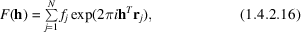whereis the diffraction vector, N is the number of atoms in the unit cell,is the atomic scattering factor including its temperature factor and depending on the magnitude of h only, andis the position vector of the jth atom referred to the origin of the unit cell.

If the crystal belongs to a point group of order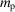and the multiplicity of its Bravais lattice is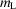, there are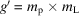general equivalent positions in the unit cell of the space group (IT A, 1983). We can thus rewrite (1.4.2.16), grouping the contributions of the symmetry-related atoms, as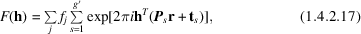whereandare the rotation and translation parts of the sth space-group operation respectively. The inner summation in (1.4.2.17)contains the dependence of the structure factor of reflection h on the space-group symmetry of the crystal and is known as the (complex) geometric or trigonometric structure factor.

Equation (1.4.2.17)can be rewritten as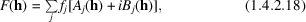whereandare the real and imaginary parts of the trigonometric structure factor. Equations (1.4.2.19)and (1.4.2.20)are mathematically identical to equations (1.4.2.11)and (1.4.2.12),respectively, apart from the numerical coefficients which appear in the expressions for A and B, for space groups with centred lattices: while only the order of the point group need be considered in connection with the Fourier expansion of the electron density (see above), the multiplicity of the Bravais lattice must of course appear in (1.4.2.19)and (1.4.2.20).

Analogous functional forms are arrived at by considerations of symmetry in direct and reciprocal spaces. These quantities are therefore convenient representations of crystallographic symmetry in its interaction with the diffraction experiment and have been indispensable in all of the early crystallographic computing related to structure determination. Their applications to modern crystallographic computing have been largely superseded by fast Fourier techniques, in reciprocal space, and by direct use of matrix and vector representations of space-group operators, in direct space, especially in cases of low space-group symmetry. It should be noted, however, that the degree of simplification of the trigonometric structure factors generally increases with increasing symmetry (see, e.g., Section 1.4.3), and the gain of computing efficiency becomes significant when problems involving high symmetries are treated with this `old-fashioned' tool. Analytic expressions for the trigonometric structure factors are of course indispensable in studies in which the knowledge of the functional form of the structure factor is required [e.g. in theories of structure-factor statistics and direct methods of phase determination (see Chapters 2.1and 2.2)].

Equations (1.4.2.19)and (1.4.2.20)are simple but their expansion and simplification for all the space groups and relevant hkl subsets can be an extremely tedious undertaking when carried out in the conventional manner. As shown below, this process has been automated by a suitable combination of symbolic and numeric high-level programming procedures.

### References

International Tables for Crystallography (1983). Vol. A, Space-Group Symmetry, edited by Th. Hahn. Dordrecht: Reidel.